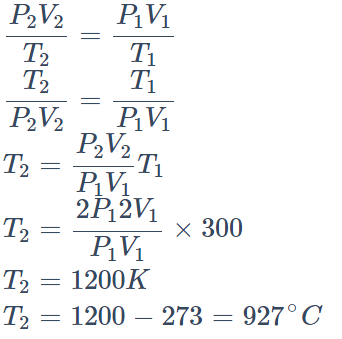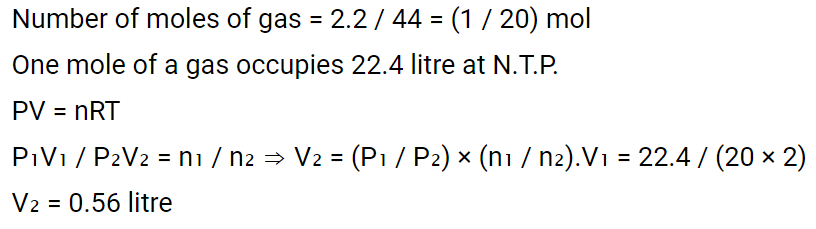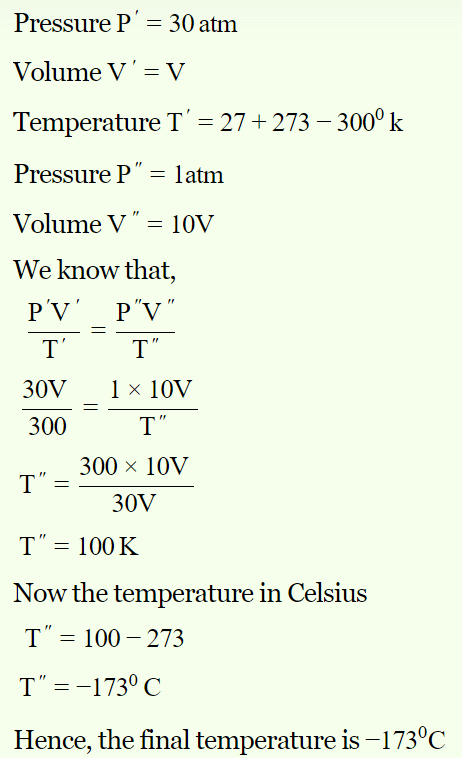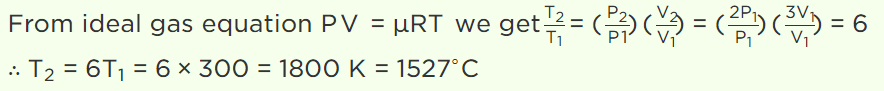## Kinetic Theory Questions and Answers Part-6

1. Kinetic theory of gases was put forward by
a) Einstein
b) Newton
c) Maxwell
d) Raman

Explanation: Kinetic theory of gases was put forward by Maxwell

2. One litre of Helium gas at a pressure 76 cm of Hg and temperature $27^{\circ}$ is heated till its pressure and volume are doubled. The final temperature attained by the gas is
a) $927^{\circ}C$
b) $900^{\circ}C$
c) $627^{\circ}C$
d) $327^{\circ}C$

Explanation:3. A flask is filled with 13 gm of an ideal gas at $27^{\circ} C$  and its temperature is raised to $52^{\circ} C$  . The mass of the gas that has to be released to maintain the temperature of the gas in the flask at $52^{\circ} C$  and the pressure remaining the same is
a) 2.5 g
b) 2.0 g
c) 1.5 g
d) 1.0 g

Explanation: Since P, V , M and R are constants,
m1T1 = m2T2
13×300=m2 ×325
m2 =12gm
Hence mass to be removed = 13-12= 1gm

4. The molecular weight of a gas is 44. The volume occupied by 2.2 g of this gas at $0^{\circ} C$  and 2 atm. pressure will be
a) 0.56 litre
b) 1.2 litres
c) 2.4 litres
d) 5.6 litres

Explanation:5. A gas at 270C temperature and 30 atmospheric pressure is allowed to expand to the atmospheric pressure. If the volume becomes 10 times its initial volume, then the final temperature becomes
a) $100^{\circ}C$
b) $173^{\circ}C$
c) $273^{\circ}C$
d) $-173^{\circ}C$

Explanation:6. When volume of system is increased two times and temperature is decreased half of its initial temperature, then pressure becomes
a) 2 times
b) 4 times
c) $\frac{1}{4} times$
d) $\frac{1}{2} times$

Explanation:7. A balloon contains 1500 m3 of helium at $27^{\circ} C$  and 4 atmospheric pressure. The value of helium at $-3^{\circ} C$  temperature and 2 atmospheric pressure will be
a) 1500 $m^{3}$
b) 1700 $m^{3}$
c) 1900 $m^{3}$
d) 2700 $m^{3}$

Explanation:8.A gas at 27°C has a volume V and pressure P. On heating its pressure is doubled and volume becomes three times. The resulting temperature of the gas will be
a) $1800^{\circ}C$
b) $162^{\circ}C$
c) $1527^{\circ}C$
d) $600^{\circ}C$

Explanation:9. The equation of state corresponding to 8 g of $O_{2}$ is
a) PV = 8RT
b) PV = RT/4
c) PV = RT
d) PV = RT/2